Related Articles

# Basic Operators in Shell Scripting

• Difficulty Level : Basic
• Last Updated : 07 Aug, 2019

There are 5 basic operators in bash/shell scripting:

• Arithmetic Operators
• Relational Operators
• Boolean Operators
• Bitwise Operators
• File Test Operators
1. Arithmetic Operators : These operators are used to perform normal arithmetics/mathematical operations. There are 7 arithmetic operators:
• Subtraction (-): Binary operation used to subtract two operands.
• Multiplication (*) :Binary operation used to multiply two operands.
• Division (/) :Binary operation used to divide two operands.
• Modulus (%) :Binary operation used to find remainder of two operands.
• Increment Operator (++) : Uniary operator used to increase the value of operand by one.
• Decrement Operator (–) : Uniary operator used to decrease the value of a operand by one
 `#!/bin/bash`` ` `#reading data from the user``read` `- p ``'Enter a : '` `a``           ``read``    ``- p ``'Enter b : '` `b`` ` `          ``add``    ``= \$((a + b))``        ``echo` `Addition of a and b are \$add`` ` `            ``sub``    ``= \$((a - b))``        ``echo` `Subtraction of a and b are \$sub`` ` `            ``mul``    ``= \$((a * b))``        ``echo` `Multiplication of a and b are \$mul`` ` `            ``div``    ``= \$((a / b))``        ``echo` `division of a and b are \$div`` ` `            ``mod``    ``= \$((a % b))``          ``echo` `Modulus of a``      ``and b are \$mod`` ` `      ``((++a))``          ``echo` `Increment``          ``operator when applied on ``"a"` `results into a = \$a`` ` `      ``((--b))``          ``echo` `Decrement``          ``operator when applied on ``"b"` `results into b = \$b`

Output: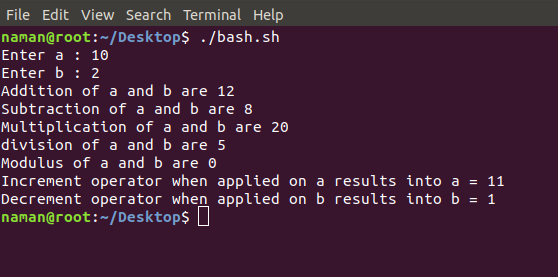2. Relational Operators : Relational operators are those operators which defines the relation between two operands. They give either true or false depending upon the relation. They are of 6 types:
• ‘==’ Operator : Double equal to operator compares the two operands. Its returns true is they are equal otherwise returns false.
• ‘!=’ Operator : Not Equal to operator return true if the two operands are not equal otherwise it returns false.
• ‘<' Operator : Less than operator returns true if first operand is lees than second operand otherwse returns false.
• ‘<=' Operator : Less than or equal to operator returns true if first operand is less than or equal to second operand otherwise returns false
• ‘>’ Operator : Greater than operator return true if the first operand is greater than the second operand otherwise return false.
• ‘>=’ Operator : Greater than or equal to operator returns true if first operand is greater than or equal to second operand otherwise returns false
 `#!/bin/bash``  ` `#reading data from the user``read` `-p ``'Enter a : '` `a``read` `-p ``'Enter b : '` `b``  ` `if``(( \$a==\$b ))``then``    ``echo` `a is equal to b.``else``    ``echo` `a is not equal to b.``fi``  ` `if``(( \$a!=\$b ))``then``    ``echo` `a is not equal to b.``else``    ``echo` `a is equal to b.``fi``  ` `if``(( \$a<\$b ))``then``    ``echo` `a is ``less` `than b.``else``    ``echo` `a is not ``less` `than b.``fi``  ` `if``(( \$a<=\$b ))``then``    ``echo` `a is ``less` `than or equal to b.``else``    ``echo` `a is not ``less` `than or equal to b.``fi``  ` `if``(( \$a>\$b ))``then``    ``echo` `a is greater than b.``else``    ``echo` `a is not greater than b.``fi``  ` `if``(( \$a>=\$b ))``then``    ``echo` `a is greater than or equal to b.``else``    ``echo` `a is not greater than or equal to b.``fi`

Output: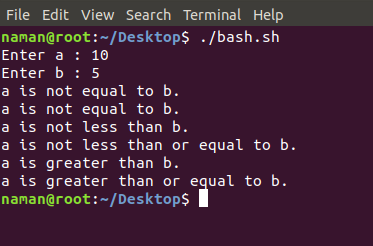3. Logical Operators : They are also known as boolean operators. These are used to perform logical operations. They are of 3 types:
• Logical AND (&&) : This is a binary operator, which returns true if both the operands are true otherwise returns false.
• Logical OR (||) : This is a binary operator, which returns true is either of the operand is true or both the operands are true and returns false if none of then is false.
• Not Equal to (!) : This is a uninary operator which returns true if the operand is false and returns false if the operand is true.
 `#!/bin/bash`` ` `#reading data from the user``read` `-p ``'Enter a : '` `a``read` `-p ``'Enter b : '` `b`` ` `if``((\$a == ``"true"` `& \$b == ``"true"` `))``then``    ``echo` `Both are ``true``.``else``    ``echo` `Both are not ``true``.``fi`` ` `if``((\$a == ``"true"` `|| \$b == ``"true"` `))``then``    ``echo` `Atleast one of them is ``true``.``else``    ``echo` `None of them is ``true``.``fi`` ` `if``(( ! \$a == ``"true"`  `))``then``    ``echo` `"a"` `was intially ``false``.``else``     ``echo` `"a"` `was intially ``true``.`` ``fi`

Output: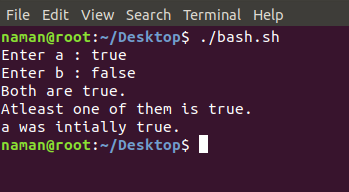4. Bitwise Operators : A bitwise operator is an operator used to perform bitwise operations on bit patterns. They are of 6 types:
• Bitwise And (&) : Bitwise & operator performs binary AND operation bit by bit on the operands.
• Bitwise OR (|) : Bitwise | operator performs binary OR operation bit by bit on the operands.
• Bitwise XOR (^) : Bitwise ^ operator performs binary XOR operation bit by bit on the operands.
• Bitwise compliment (~) : Bitwise ~ operator performs binary NOT operation bit by bit on the operand.
• Left Shift (<<) : This operator shifts the bits of the left operand to left by number of times specified by right operand.
• Right Shift (>>) : This operator shifts the bits of the left operand to right by number of times specified by right operand.
 `#!/bin/bash`` ` `#reading data from the user``read` `-p ``'Enter a : '` `a``read` `-p ``'Enter b : '` `b`` ` `bitwiseAND=\$(( a&b ))``echo` `Bitwise AND of a and b is \$bitwiseAND`` ` `bitwiseOR=\$(( a|b ))``echo` `Bitwise OR of a and b is \$bitwiseOR`` ` `bitwiseXOR=\$(( a^b ))``echo` `Bitwise XOR of a and b is \$bitwiseXOR`` ` `bitiwiseComplement=\$(( ~a ))``echo` `Bitwise Compliment of a is \$bitiwiseComplement`` ` `leftshift=\$(( a<<1 ))``echo` `Left Shift of a is \$leftshift`` ` `rightshift=\$(( b>>1 ))``echo` `Right Shift of b is \$rightshift`

Output: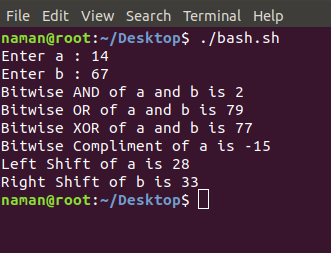5. File Test Operator : These operators are used to test a particular property of a file.
• -b operator : This operator check weather a file is a block special file or not. It returns true, if the file is a block special file otherwise false.
• -c operator : This operator checks weather a file is a character special file or not. It returns true if it is a character special file otherwise false.
• -d operator : This operator checks if the given directory exists or not. If it exits then operators returns true otherwise false.
• -e operator : This operator checks weather the given file exits or not. If it exits this operator returns true otherwise false.
• -r operator : This operator checks weather the given file has read access or not. If it has read access then it returns true otherwise false.
• -w operator : This operator check weather the given file has write access or not. If it has write then it returns true otherwise false.
• -x operator : This operator check weather the given file has execute access or not. If it has execute access then it returns true otherwise false.
• -s operator : This operator checks the size of the given file. If the size of given file is greater than 0 then it returns true otherwise it false.
 `#!/bin/bash`` ` `#reading data from the user``read -p ``'Enter file name : '` `FileName`` ` `if` `[ -e \$FileName ]``then``    ``echo File Exist``else``    ``echo File doesnot exist``fi`` ` `if` `[ -s \$FileName ]``then``    ``echo The given file is not empty.``else``    ``echo The given file is empty.``fi`` ` `if` `[ -r \$FileName ]``then``    ``echo The given file has read access.``else``    ``echo The given file does not has read access.``fi`` ` `if` `[ -w \$FileName ]``then``    ``echo The given file has write access.``else``    ``echo The given file does not has write access.``fi`` ` `if` `[ -x \$FileName ]``then``    ``echo The given file has execute access.``else``    ``echo The given file does not has execute access.``fi`

Output: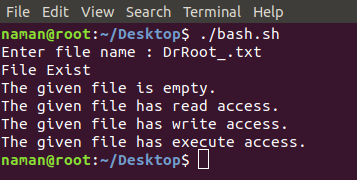My Personal Notes arrow_drop_up